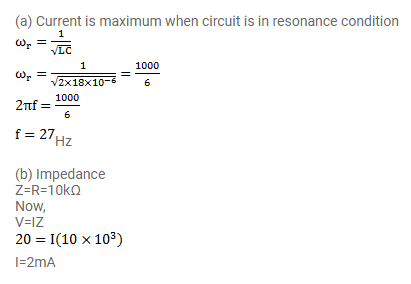# An inductance of 2.0H,

Question:

An inductance of $2.0 \mathrm{H}$, a capacitance of $18 \mu \mathrm{F}$ and a resistance of $10 \mathrm{k} \Omega$ are connected to an Ac source of $20 \mathrm{~V}$ with adjustable frequency.

(a) What frequency should be chosen to maximize the current in the circuit?

(b) What is the value of this maximum current?

Solution: